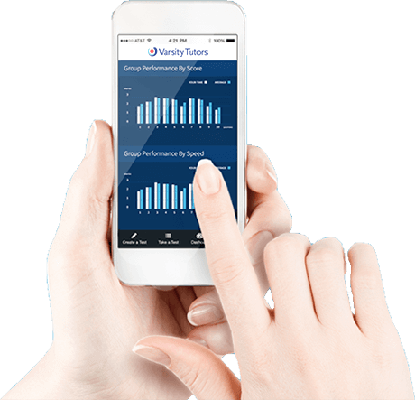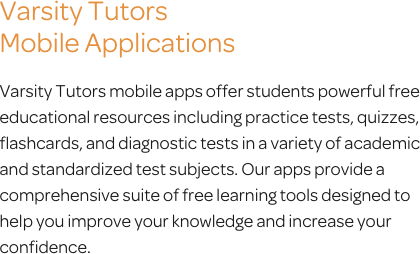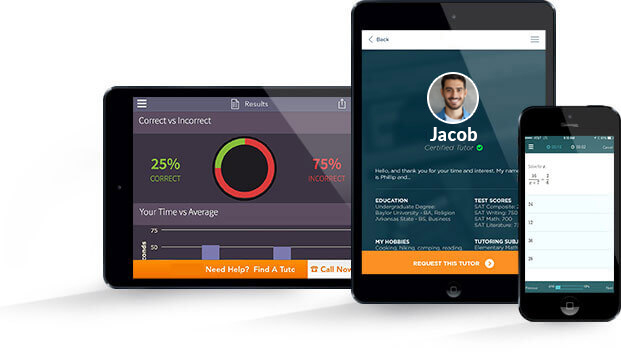# Varsity Tutors Trigonometry mobile app### The Varsity Tutors Trigonometry Mobile App

Trigonometry, as you might be able to guess, relates to the study of triangles. It’s no longer a matter of simply solving equations, as you will be working on the relationships of the sides and angles of triangles. You will now have to use spatial reasoning, which may seem difficult as you begin learning this new mathematical approach.

Fortunately, regular practice is one of the best ways to achieve a deeper understanding of trigonometry. The free Varsity Tutors Trigonometry app for iPhone, iPad, and Android devices is an on-the-go learning tool suitable for all trigonometry students. With comprehensive content in the form of practice tests, flashcards, and more, the app is a perfect study aid.

On the app, you’ll find all of the concepts you’ll need to know in trigonometry. First, you will learn about angles: complementary, supplementary, and coterminal. You will also look at angles in different quadrants.

Then, you will learn about triangles. First, the laws of sine and cosine -- the two main laws of trigonometry -- are explained in depth. Skills related to right triangles follow. You will learn how to find the values of triangles’ angles and sides when you know some, but not all, of the information about them.

Next, you will dive into trigonometric equations. Here, you will learn how to understand and solve trigonometric roots, how the quadratic formula is used in trigonometry, and the systems of trigonometric equations. Trigonometric functions and graphs are also covered, including how to simplify trigonometric functions, and how to graph the secant and cosecant, sine and cosine, and tangent and cotangent. You will also get into period, amplitude, and phase shifts.

In trigonometric identities, you will learn about basic, definitional, complementary, and supplementary identities. You will learn about the identities of doubled angles, halved angles, inverse operations, squared trigonometric functions, and angle sums. You will also learn the ubiquitous Pythagorean identity.

The app then reviews major trigonometric operations, including arcsin, arccos, arctan, sec, csc, ctan, sin, cos, and tan. Finally, you will be taken through unit circles and radians, including angles in the unit circle, and radians and conversions.

You can use one of the full-length, timed trigonometry practice tests to discover your strengths and weaknesses. On every practice test, you will receive comprehensive feedback with a variety of useful metrics, like your solving time for each question, concepts you should review, and more. For each question, the test feedback provides not only the correct answer, but an in-depth explanation of the steps you must take to reach that answer. After you review the areas you need work in, you can retake the practice tests. The app keeps track of all your scores, so you can watch your progress.

Once you know which areas need review, you can use Learn by Concept or the flashcards to dig into specific topics, such as how to identify similar triangles. You can even make your own flashcards for a personalized learning experience! The Flashcard Maker allows you to use your own pictures, text, and audio to create a custom set of cards.

Trigonometry is a tough subject, but you can give yourself a great chance for success by downloading the free Varsity Tutors Trigonometry app from iTunes or the Google Play Store today!

Once you know which areas need review, you can use Learn by Concept or the flashcards to dig into specific topics, such as how to identify similar triangles. You can even make your own flashcards for a personalized learning experience! The Flashcard Maker allows you to use your own pictures, text, and audio to create a custom set of cards.

Trigonometry is a tough subject, but you can give yourself a great chance for success by downloading the free Varsity Tutors Trigonometry app from iTunes or the Google Play Store today!Trigonometry is a discipline of math that focuses on the measurements, properties, and relationships of triangles. There are high school and college-level courses that use trigonometry. In fact, trigonometry plays a critical role in developing more advanced mathematical skills. It can be helpful when working in certain majors, such as accounting, social work, psychology, economics, business management, and mathematics. Taking the program during high school can potentially help you test out of requirements, save money on tuition, and speed up your progression in your first year of college.

At the end of the school year, you will take a trigonometry final exam that covers the concepts that will be introduced and reinforced during the course. The exam will require that you recall the various formulas, equations, and theories necessary to solve a wide range of different problems. While much of this may be memorization, the actual skill and knowledge in assessing, analyzing, and comprehending data and other numerical information is crucial to successfully completing the course exam. The exam is typically given during a class period at your high school. You will find questions that require you to solve for the angle sum or difference, determine the correct trigonometric formula to use, predict the ending point for a graph, or create your own graphs to back up your answers. In addition, you will be tested on your ability to identify angles, as well as your knowledge of co-function relationships and understanding of the unit circle.

When you take trigonometry, you will learn a variety of triangle-related concepts. You will learn about trigonometric ratios, how to perform operations using the six ratios, how to develop an understanding for the different types of identities, and learn about the many real-world applications of trigonometry. Also, you will learn about the special values for 3 through 6 pi, how to solve special triangle cases, how to find the inverse of a trigonometric function through cyclometric functions, and about angle sum and difference.

The first section of the course focuses on developing a deeper understanding of angles and how they relate to various formulas. Attention is placed on complementary and supplementary angles, coterminal angles, and the placement of angles in different quadrants on a graph based on their characteristics. You will be introduced to locating angles based on reference and rotations, as well as how to make conversions from radians to degrees and back. You will learn to evaluate a wide range of angles to prepare you for topics on triangles.

The second portion of a high school trigonometry class focuses on the basics of triangles and trigonometry. During this section, you review the various types of triangles and their characteristics, measuring angles and sides, and rules of proportion in similar triangles. You’ll cover identifying right-angle relationships that can help you choose the proper trigonometric function. In addition, you'll learn about the law of cosines, law of sines, and Heron's formula. By the end of this section, you should be capable of explaining the various laws that were discussed, how they are implemented differently depending on whether you’re working with a right triangle or otherwise, and how to determine the formulas needed to solve specific problems.

During the third portion of your trigonometry class, you will cover more advanced trigonometric functions. By the end of this section, you will be capable of identifying the correct usage for tangent, cosine, and sine; graphing the different functions; using horizontal and vertical translations based on their specific rules; and transforming trigonometric functions with compression and stretching. In addition, your instructor will spend some time going over the concept of reciprocal functions to help you learn to differentiate between cosecant, secant, and other functions. Furthermore, you will learn to graph, evaluate, define, and recognize them, along with their corresponding inverses.

The next main principle you learn in this course will be trigonometric equations. The class introduces you to each one to allow you to learn the rules for each type of equation. You will need to grasp the principle of trigonometric identities, as they are required to solve many trigonometric equations. You will have to understand how to identify and distinguish sums, double-angles, Pythagorean, half-angle, and difference identities in order to successfully solve each equation. You will cover the base equations for cosine, tangent, cotangent, and sine, as well as how you can reduce these equations, use factoring alongside them, and use additional unknown variables.

The final section of trigonometry covers radians and unit circles. The unit circle is an introductory point to changing your thinking of trigonometry from triangles to arbitrary real numbers. Your teacher will expect you to form an understanding of the relationship between degrees and radians, how they express angles, and how you can convert the measurements from one to the other. You will need to be able to use the appropriate formulas to find the arc length of the unit circle, identify the appropriate trigonometric function to use for various types of angles, and how to transfer these functions onto a graph. You'll learn how to differentiate between periodic functions with a period of 1, 2 or 3 pi. You will be required to demonstrate the ability to graph these periodic functions as well. In addition, you will learn how to apply these functions to special triangles with unique angles and how to combine different identities together.

Trigonometry is a math course that can open doors for you. If you take trigonometry, you may be able to successfully complete the SAT Subject Test in Math II, which can look incredible to an admissions officer at your school of choice. In addition, you can save money on your classes and be prepared for more advanced courses by getting trigonometry taken care of early on. The course has a heavy focus on triangles, which may seem like an easy concept to master at first glance, but many students find that memorizing the different types of equations they need to be able to recall and apply properly on exams is no easy task. That's where additional study and preparation come into play.